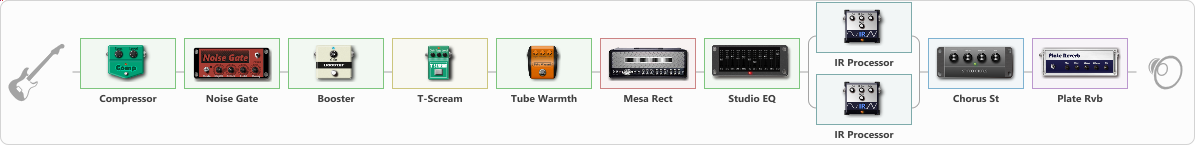# best Mesa preset

Discussion in 'ToneLib-GFX presets' started by Tony the Metalhead, May 31, 2022.

1. ### Tony the MetalheadActive Member

best Mesa preset

Preset name: MESA, but not a BOOGIE

Effects chain:Effect: "Compressor" (Dynamics / Filter), active - "yes"
{
"Sense" = 40
"Level" = 25
}

Effect: "Noise Gate" (Dynamics / Filter), active - "yes"
{
"Mode" = Auto
"Depth" = 50
"Threshold" = 50
"Attack" = 0
"Hold" = 5
"Decay" = 250
}

Effect: "Booster" (Dynamics / Filter), active - "yes"
{
"Gain" = 25
}

Effect: "T-Scream" (Overdrive / Distortion), active - "yes"
{
"Drive" = 5
"Tone" = 80
"Level" = 40
}

Effect: "Tube Warmth" (Dynamics / Filter), active - "yes"
{
"Input" = 45
"Warmth" = 60
"Level" = 100
}

Effect: "Mesa Rect" (Amp simulators), active - "yes"
{
"Gain" = 55
"Bass" = 60
"Middle" = 0
"Treble" = 80
"Presence" = 55
"Master" = 40
"Level (dB)" = 5
}

Effect: "Studio EQ" (Dynamics / Filter), active - "yes"
{
"31 Hz" = -3
"62 Hz" = -2
"125 Hz" = -4
"250 Hz" = -6
"500 Hz" = -5
"1 kHz" = -4
"2 kHz" = 4
"4 kHz" = 8
"8 kHz" = 8
"16 kHz" = 10
"Level (dB)" = 0
}

Effect: "Splitter" (Dynamics / Filter)
{
"A-Bypass" = Off
"A-Pan" = -25
"A-Level" = 55
"B-Bypass" = Off
"B-Pan" = 25
"B-Level" = 55
"Width" = 25

'A' branch:
{

Effect: "IR Processor" (Cabinets), active - "yes"
{
"IR" = RKH 4x12 V30 SHR SM57 Center
"Low Cut (Hz)" = 100
"Hi Cut (kHz)" = 12.0
"Mix" = 100
"Level (dB)" = -20
}
}
'B' branch:
{

Effect: "IR Processor" (Cabinets), active - "yes"
{
"IR" = RKH 4x12 V30 SHR SM57 Off axis 2
"Low Cut (Hz)" = 140
"Hi Cut (kHz)" = 18.0
"Mix" = 100
"Level (dB)" = 0
}
}
}

Effect: "Chorus St" (Modulation / Sfx), active - "yes"
{
"Speed" = 0.1
"Depth" = 22
"Center" = 8.0
"Mix" = 42
}

Effect: "Plate Rvb" (Reverberation), active - "yes"
{
"Time" = 6.0
"PreDelay" = 0
"LoDamp" = 0
"HiDamp" = 100
"Mix" = 40
}

Note: You will need to download and install the ToneLib-GFX software to use the preset.

File size:
11.4 KB
Views:
1,863